Subtotal function in Excel

The SUBTOTAL function ignores any rows that are not included in the result of a filter, no matter which function_num value you use. The SUBTOTAL function is designed for columns of data, or vertical ranges.

Use the SUBTOTAL function in Excel instead of SUM, COUNT, MAX, etc. to ignore rows hidden by a filter or manually hidden rows.

Rows Hidden by a Filter

1. For example, the SUM function below calculates the total sales.

Also See:   Understanding Named Range, Named Constant, Name Manager in Excel Formulas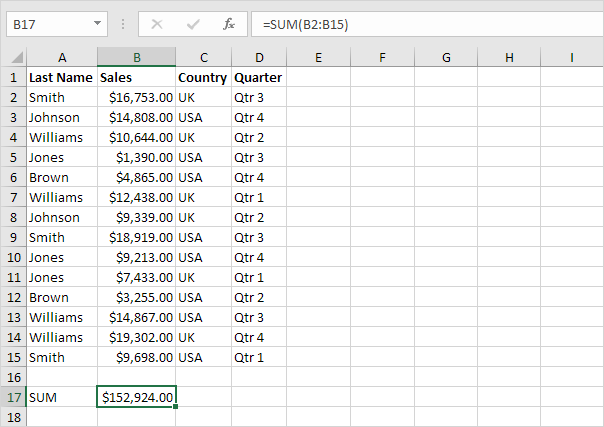2. Apply a filter.

3. The SUM function includes rows hidden by a filter.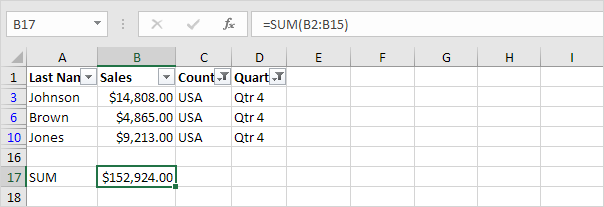4. The SUBTOTAL function ignores rows hidden by a filter and calculates the correct result.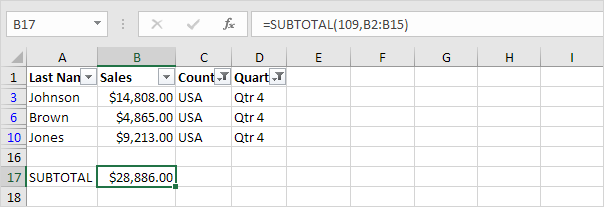Note: 109 is the argument for Sum if you use the SUBTOTAL function.

5. It’s not easy to remember which argument belongs to which function. Fortunately, the AutoComplete feature in Excel helps you with this.

Also See:   Dynamic Named Range in Excel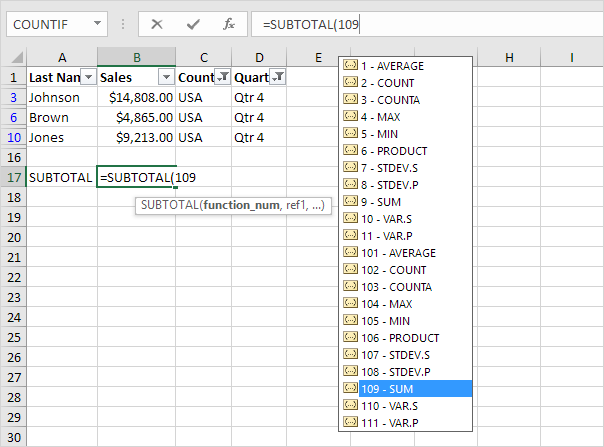Note: instead of using 101 (AVERAGE) for the first argument, you could also use 1 (AVERAGE), instead of using 102 (COUNT), you could also use 2 (COUNT), etc. When filtering data, there’s no difference between the numbers 101-111 and the numbers 1-11. We recommend using the numbers 101-111 because Excel uses these numbers in total rows at the end of tables.

Manually Hidden Rows

1. For example, the SUM function below sums a range of cells.

Also See:   How to calculate percentage of total in Excel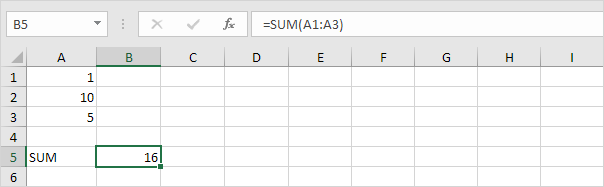2. Hide row 2.

3. The SUM function includes manually hidden rows.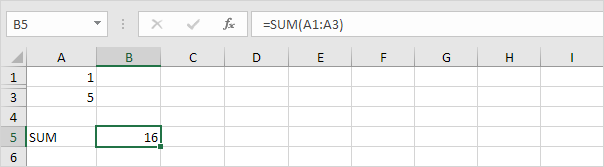4. The SUBTOTAL function ignores manually hidden rows and calculates the correct result.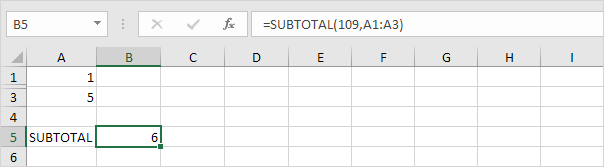Note: when hiding rows, the numbers 101-111 ignore manually hidden rows (step 4). The numbers 1-11 include manually hidden rows but remember, SUM, COUNT, MAX, etc. give the exact same result (step 3).